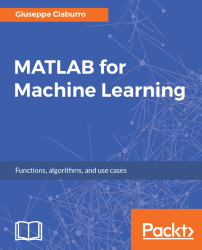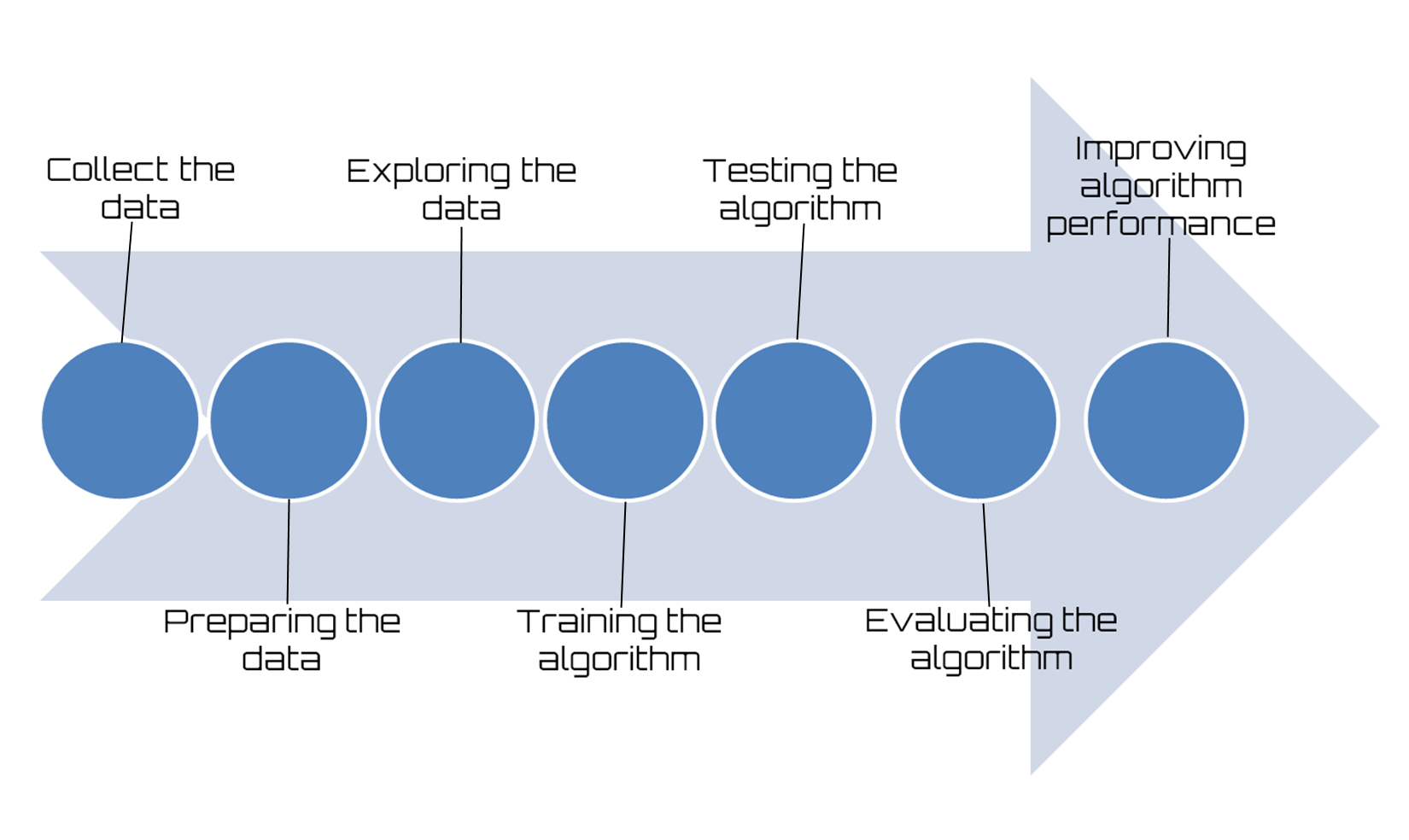•#### MATLAB for Machine Learning#### Overview of this book

MATLAB is the language of choice for many researchers and mathematics experts for machine learning. This book will help you build a foundation in machine learning using MATLAB for beginners. You’ll start by getting your system ready with t he MATLAB environment for machine learning and you’ll see how to easily interact with the Matlab workspace. We’ll then move on to data cleansing, mining and analyzing various data types in machine learning and you’ll see how to display data values on a plot. Next, you’ll get to know about the different types of regression techniques and how to apply them to your data using the MATLAB functions. You’ll understand the basic concepts of neural networks and perform data fitting, pattern recognition, and clustering analysis. Finally, you’ll explore feature selection and extraction techniques for dimensionality reduction for performance improvement. At the end of the book, you will learn to put it all together into real-world cases covering major machine learning algorithms and be comfortable in performing machine learning with MATLAB.
Title PageCreditsForewordwww.PacktPub.comCustomer FeedbackPrefaceFree Chapter
Getting Started with MATLAB Machine LearningImporting and Organizing Data in MATLABFrom Data to Knowledge DiscoveryFinding Relationships between Variables - Regression TechniquesPattern Recognition through Classification AlgorithmsIdentifying Groups of Data Using Clustering MethodsSimulation of Human Thinking - Artificial Neural NetworksImproving the Performance of the Machine Learning Model - Dimensionality ReductionMachine Learning in Practice## How to build machine learning models step by step

Finally, the algorithm to apply to our data is chosen; it is now time to get down to work without delay. Before you tackle such a job, it is appropriate to devote some time to the workflow setting. When developing an application that uses machine learning, we will follow a procedure characterized by the following steps:

1. Collect the data: Everything starts from the data, no doubt about it; but one might wonder where so much data comes from. In practice, it is collected through lengthy procedures that may, for example, derive from measurement campaigns or face-to-face interviews. In all cases, the data is collected in a database so that it can then be analyzed to derive knowledge.

### Note

If we do not have specific requirements, and to save time and effort, we can use publicly available data. In this regard, a large collection of data is available in the UCI Machine Learning Repository at the following link: http://archive.ics.uci.edu/ml.

The following figure shows how to build machine learning models step by step:Figure 1.10: Machine learning workflow

1. Preparing the data: We have collected the data; now we have to prepare it for the next step. Once we have this data, we must make sure it is in a format usable by the algorithm we want to use. To do this, you may need to do some formatting. Recall that some algorithms need data in an integer format, whereas others require data in the form of strings, and finally others need to be in a special format. We will get to this later, but the specific formatting is usually simple compared to data collection.
2. Exploring the data: At this point, we can look at data to verify that it is actually working and we do not have a bunch of empty values. In this step, through the use of plots, we can recognize patterns or whether there are some data points that are vastly different from the rest of the set. Plotting data in one, two, or three dimensions can also help.
3. Training the algorithm: Now let's get serious. In this step, the machine learning begins to work with the definition of the model and the next training. The model starts to extract knowledge from large amounts of data that we had available, and that nothing has been explained so far. For unsupervised learning, there’s no training step because you don’t have a target value.
4. Testing the algorithm: In this step, we use the information learned in the previous step to see if the model actually works. The evaluation of an algorithm is for seeing how well the model approximates the real system. In the case of supervised learning, we have some known values that we can use to evaluate the algorithm. In unsupervised learning, we may need to use some other metrics to evaluate success. In both cases, if we are not satisfied, we can return to the previous steps, change some things, and retry the test.
5. Evaluating the algorithm: We have reached the point where we can apply what has been done so far. We can assess the approximation ability of the model by applying it to real data. The model, preventively trained and tested, is then valued in this phase.
6. Improving algorithm performance: Finally we can focus on the finishing steps. We've verified that the model works, we have evaluated the performance, and now we are ready to analyze the whole process to identify any possible room for improvement.